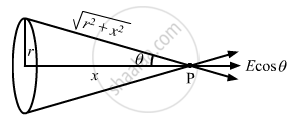Advertisement Remove all ads

# Consider a Circular Ring of Radius R, Uniformly Charged with Linear Charge Density λ. Find the Electric Potential at a Point on the Axis at a Distance X from the Centre of the Ring - Physics

Numerical

Consider a circular ring of radius r, uniformly charged with linear charge density λ. Find the electric potential at a point on the axis at a distance x from the centre of the ring. Using this expression for the potential, find the electric field at this point.

Advertisement Remove all ads

#### Solution

Given:
Radius of the ring = r
So, circumference = 2πr
Charge density = λ,
Total charge, q = 2πr × λ
Distance of the point from the centre of the ring = x
Distance of the point from the surface of the ring,

$r' = \sqrt{r^2 + x^2}$
Electricity potential,
$V = \frac{1}{4\pi \epsilon_0}\frac{q}{r'}$
$\Rightarrow V = \frac{1}{4\pi \epsilon_0}\frac{2\pi r\lambda}{\sqrt{r^2 + x^2}}$

$\Rightarrow V = \frac{1}{2 \epsilon_0}\frac{r\lambda}{( r^2 + x^2 )^{1/2}}$Due to symmetry at point P, vertical component of electric field vanishes.
So, net electric field = Ecosθ

$\Rightarrow E = \frac{r\lambda}{2 \epsilon_0 ( r^2 + x^2 )^{1/2}}\frac{x}{( r^2 + x^2 )}$
$\Rightarrow E = \frac{r\lambda x}{2 \epsilon_0 ( r^2 + x^2 )^{3/2}}$

Concept: Electric Field - Introduction of Electric Field
Is there an error in this question or solution?
Advertisement Remove all ads

#### APPEARS IN

HC Verma Class 11, Class 12 Concepts of Physics Vol. 2
Chapter 7 Electric Field and Potential
Q 63 | Page 123
Advertisement Remove all ads
Advertisement Remove all ads
Share
Notifications

View all notifications

Forgot password?